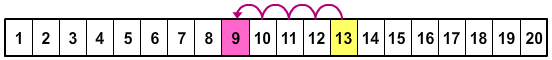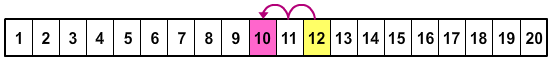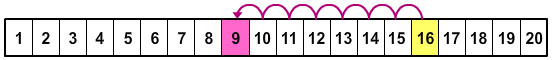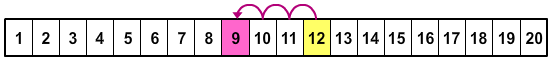# Ways to Make a Number Using Subtraction Part 1

Rate 0 stars Common Core
Quiz size:
Message preview:
Someone you know has shared Ways to Make a Number Using Subtraction Part 1 quiz with you:

To play this quiz, click on the link below:

https://www.turtlediary.com/quiz/ways-to-make-a-number-using-subtraction.html

Hope you have a good experience with this site and recommend to your friends too.

Login to rate activities and track progress.
Login to rate activities and track progress.

Consider the following options:

13 – 4

12 – 2

16 – 7

12 – 3

Let's identify all the correct ways to make number 9.

First check the difference of given numbers in each option by counting back from the bigger number.

Consider the first option.

13 – 4 = ?

Starting from 13, count back 4.So, 13 – 4 = 9

Now, check the second option.

12 – 2 = ?

Starting from 12, count back 2.So, 12 – 2 = 10

Now, check the third option.

16 – 7 = ?

Starting from 16, count back 7.So, 16 – 7 = 9

Now, check the last option.

12 – 3 = ?

Starting from 12, count back 3.So, 12 – 3 = 9

So, the correct ways to make number 9 are 13 – 4, 16 – 7, and 12 – 3.

ds

A B C D E F G H I J K L M N O P Q R S T U V W X Y Z